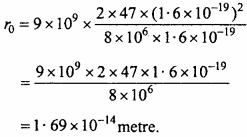## MP Board Class 12th Physics Important Questions Chapter 12 Atoms

### Atoms Important Questions

Atoms Objective Type Questions

Question 1.
Choose the correct answer of the following:

Question 1.
According to Ruther ford’s atomic model the electrons inside the atom are:
(a) Stationary
(b) Centralized
(c) Revolving
(d) None of these.
(c) Revolving

Question 2.
The size of atom is of order of:
(a) 10-15 m
(b) 10-15 cm
(c) 10-10 m
(d) 10-10 cm.
(c) 10-10 m

Question 3.
In Bohr’s model in an stable orbit the speed of electron vn is related with principle quantum number as:
(a) vn = $$\frac {c}{n}$$
(b) v = $$\frac {n}{c}$$
(c) v = n.c
(d) $$\frac { c }{ { n }^{ 2 } }$$
(a) vn = $$\frac {c}{n}$$Question 4.
In terms of Rydberg constant R the wave number of first spectral line of Balmar series is :
(a) R
(b) 3R
(c) $$\frac {5R}{18}$$
(d) $$\frac {8R}{9}$$
(c) $$\frac {5R}{18}$$

Question 5.
Hydrogen atom is excited from ground state to n = 4. The number of spectral lines observed be:
(a) 3
(b) 6
(c) 5
(d) 2
(b) 6

Question 6.
The value of Bohr’s radius is :
(a) 5.3 x 10-11 nm
(b) 3.5 x 10-10 m
(c) 53.3 x 10-11 nm
(d) None of these.
(a) 5.3 x 10-11 nm

Question 7.
The value of Rydberg constant R is :
(a) 1.097 x 10-11 nm
(b) 1.097 x 10-8 m-1
(c) 1.097 x 108 m-1
(d) 1.097 x 107 m-1.
(d) 1.097 x 107 m-1.

Question 8.
According to Bohr the speed of electron of first orbit is :
(a) $$\frac {137}{c}$$
(b) 137 x c
(c) 137 + c
(d) $$\frac {c}{137}$$
(d) $$\frac {c}{137}$$

Question 2.
Fill in the blanks :

1. In the Geiger Marsdan’s α – particle experiment the energy of a particle was ……………
2. According to Rutherford’s atomic model the total energy of energy was …………… (Positive/Negative)
3. The spectral lines of visible region of hydrogen spectrum was first seen by ……………
4. The value of Rydberg constant R is ……………
5. The electron revolve round the nucleus in such orbits in which the value of angular momentum is integral multiple of ……………
6. The maximum ionisation energy of hydrogen atom is ……………
7. In first excited state of hydrogen atom the total energy of electron is about …………… eV.

1. 5.5 MeV
2. Negative
3. Balmar
4. 1.097 x 107m-1
5. $$\frac {h}{2π}$$
6. 13.6 MeV
7. -3.4 eV.

Question 3.
Match the column: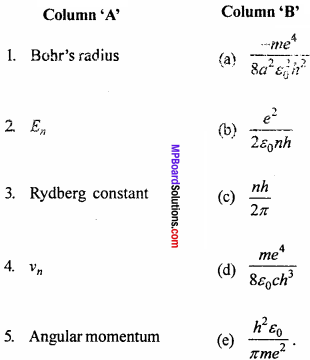1. (e)
2. (a)
3. (d)
4. (b)
5. (c)

Question 4.
Write the answer in one word/sentence:

1. What is α – pariide?
2. Write an important conclusion of Thomson’s atomic model.
3. Which important part of the atom was discovered by Rutherford?
4. In which condition the Balmar spectral line in the hydrogen spectrum is obtained?
5. What is the order of radius of nucleus of an atom?
6. What is order of size of atom?
7. Which of the following particle is unstable? α – particle, β – particles, protons, neutrons.

1. The helium nucleus 2He4 is called as α – particle
2. The mass of atom and positive charge is uniformly distributed in the sphere
3. Nucleus
4. When the electrons jump from higher energy level to second energy level
5. 10-14 m
6. 10-10 m
7. Neutrons.

Atoms Very Short Answer Type Questions

Question 1.
What will be the value of scattering angle of α – par tide if impact parameter is zero?
In the relation b ∝ cot$$\frac {θ}{2}$$ then
cot$$\frac {θ}{2}$$ = 0
or $$\frac {θ}{2}$$ = 90°
or θ = 180°

Question 2.
In Bohr’s model the radius of first orbit is π0. What will be the radius of second orbit?
From the formula πn = π0 n2
If n = 2
Then π2 = π0 x 22 = 4π0Question 3.
Define stable orbit in Bohr’s atoms model.
The stable orbits are those orbits in which the angular momentum of the electron (revolving in those orbits) is integral multiple of $$\frac {h}{2π}$$

Question 4.
State Bohr’s quantization condition.
Angular momentum mvr = $$\frac {nh}{2π}$$, where n = 1,2,3 …..

Question 5.
Write quantization condition for the angular momentum of electron revolving in second orbit.
In the formula mvr = $$\frac {nh}{2π}$$
If n = 2
Then mvr = $$\frac {2h}{2π}$$ = $$\frac {h}{π}$$

Question 6.
What is importance of negative energy of electrons in an orbit?
The nucleus and electrons makes a bounded system together.

Question 7.
What would happen if the electrons were at rest inside the atom?
If the electrons were stationary inside the atom, then they tends to attract to – wards the nucleus.

Question 8.
Why the electrons always revolve round the nucleus?
In order to provide a stable configuration to the atoms.

Question 9.
What is Bohr’s radius?
The radius of first orbit of a hydrogen atom is called Bohr’s radius. Its value is 0.53 Å.

Atoms Short Answer Type Questions

Question 1.
Why only gold leaf is taken for the scattering of α – particles?
For the scattering of α – particles, the leaf should be very thin, so that α – particles gets scattered after collision. Moreover the nucleus should be heavy, so that the α – particles are scattered by large angles. Gold has both the properties moreover this foil of gold can easily be made.

Question 2.
What is angular momentum of electron revolving in first Bohr’s orbit?
The angular momentum in nth orbit is
mvr = $$\frac {nh}{2π}$$
Put n = 1
The angular momentum in first orbit = $$\frac {h}{2π}$$.

Question 3.
The spectrum of hydrogen atom has many lines though a hydrogen atom contains only one electron why?
Large number of spectral lines are present in hydrogen atom spectrum because in the light source of hydrogen a large number of atoms are there. There is different transitions in different atoms. That is why hydrogen spectrum contains number of lines.

Question 4.
What is meant by transition? How much time it takes?
The jump of an atom from one energy state (level) to other is called transition. It takes about 10-8 sec.Question 5.
What may be the maximum and minimum number of emission transitions between energy levels n = 5 to n = 1.
Maximum numbers = 10
(5 → 4, 5 → 3, 5 → 2, 5 → 1, 4 → 3, 4 → 2, 4 → 1, 3 → 2, 3 → 1, 2 →1)
Minimum numbers = 1 (5 → 1).

Question 6.
What will happen if electron remain stationary in an orbit?
If the electron remains stationary in an orbit it would fall into the nucleus due to electrostatic attraction of nucleus. So the atom would be unstable.

Question 7.
With the help of fundamental constants e, me and h establish a quantity in the dimension of length.
This quantity is Bohr’s radius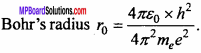Question 8.
If the elements with principal quantum number n > 4 are not included in nature then what will be the number of such possible elements?
For principal quantum n = 4 the atom will have only K, L, M and N shell. The maximum number of electrons in these shell according to 2n2 will be, 2, 8 18 and 32 i.e., the maximum number of electrons in these shell will be 2 + 8 – 18 – 32 = 60. Thus the number of such elements be 60.

Question 9.
What is the significance of the negative energy of electron in the orbit? If it be positive then what does it mean?
Total energy of electron E = K + U = $$\frac { { -e }^{ 2 } }{ 8\pi { \varepsilon }_{ 0 }r }$$
Here negative sign shows that the electron is bounded with nucleus if the negative energy be positive then it means that the electron will not revolve round the nucleus in a bounded orbit.

Question 10.
Bohr’s orbit is called stable orbit. Why?
Bohr’s orbit is called as stable orbit because the electron revolving in this orbit neither emit the energy nor absorb.

Question 11.
The nuclear density is more than that of atom. Why?
we know that density = $$\frac {Mass}{Volume}$$.
The mass of nucleus and atoms are almost same but the radius of nucleus is about of order of 10-15 m and that of atom is about of order of 10-10 m. Hence the volume of nucleus is of order of 10-45 m3 and that of atom is of order of 10-30 m3. Obviously the volume of nucleus is about 10-15 times that of atom, so the nuclear density is more than that of atom.

Question 12.
What are Bohr’s quantum condition and frequency conditions?
1. The electrons can revolve in those orbits in which the angular momentum of electron is integral multiple of $$\frac {h}{2π}$$. Where h is Planck’s constant i.e.
Angular momentum L = mvr = $$\frac {nh}{2π}$$, where n = 1,2,3, …..
This is called as Bohr’s quantum condition.

2. When an electron jumps from energy level of higher energy E2 to the energy level having energy E1 then a photon is emitted whose energy is equal to the difference of energies of the two levels i.e., E2 – E1 = hu.
Where o is frequency of emitted photon. This is called Bohr’s frequency condition.

Question 13.
Calculate the radius of first orbit of hydrogen atom. Prove that the velocity of electron in first orbit is $$\frac {1}{137}$$ times the velocity of light. (NCERT)
Solution: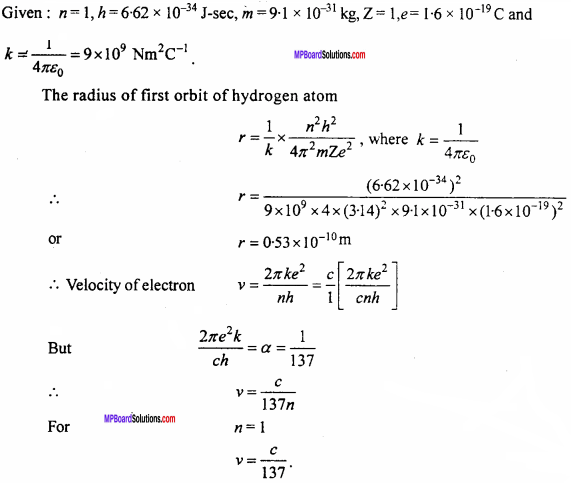Question 14.
According to the equation $$\frac {1}{λ}$$ = R($$\frac { 1 }{ { n }_{ 2 }^{ 2 } } -\frac { 1 }{ { n }_{ 1 }^{ 2 } }$$),
what will be the shortest wavelength which will either be absorbed or be emitted?
Solution:
For the shortest wavelength the R.H.S. of the equation should be greatest i.e.,
when n1 = ∞ and n2 = 1, then $$\frac {1}{λ}$$ = R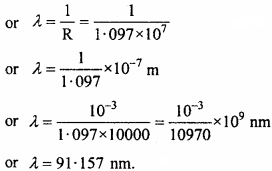Question 15.
Explain the Bohr’s quantization condition of angular momentum.
Bohr’s quantization condition: The electron can revolve only in those orbits in which the angular momentum of electron is integral multiple of $$\frac {h}{2π}$$ and h is Planck’s constant.

Atoms Long Answer Type Questions

Question 1.
Describe Rutherford’s experiment related to α – particles scattering. Also write conditions drawn from it
The experimental arrangement of Rutherford’s α – particles scattering experiment is shown in fig. A radioactive substance radon as ‘Po’ is kept in a lead box from the hole ‘O’ the α – particles are emitted out with high speed. After passing through D1 and D2 these α – particles are incident on gold leaf in the form of a beam.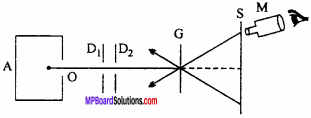The thickness of gold , foil is about 10 cm. The scattered α – particles falls on screen S in which ZnS is coated α – particles produce flash of light on this screen which can be observed by a microscope M.

Conclusion:

1. Most of the α – particles pass through the foil straight undeflected. Thus it can be concluded that most of the part of atom is hollow.
2. α – particles are repelled so, there should be positive charge on nucleus.
3. The entire positive charge should be on the centre of atom (or nucleus).

Importance:
By the Rutherford’s experiment it is come to know that the positive charge of the atom is concentrated at the central core of atom, which is called as nucleus.Question 2.
The total energy of an electron in the first excited state of the hydrogen atom is about -3.4 eV :

1. What is the kinetic energy of the electron in this state?
2. What is the potential energy of the electron in this state?
3. Which of the answers above would change, if the choice of the zero of potential energy is changed?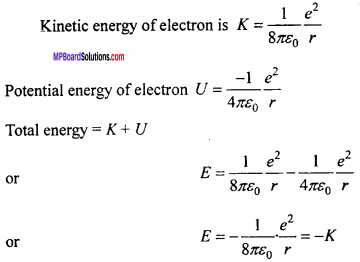Also U = – 2 K

1. Here £ = – 3.4 eV
Thus K = – E = -(-3.4) eV
= 3.4 eV.

2. Here U = – 2K
= – 2 (3.4) eV
= – 6.8 eV.

3. E = – 3.4 eV only when the zero of potential energy is at infinity. If the zero of the potential energy is changed, only potential energy (hence total energy) would change. However kinetic energy remain unaffected.

Question 3.
Prove that the radius of nth Bohr’s orbit of an atom is proportional to nth, where n is principal quantum number.
The angular momentum of electron in n stable orbit is integral multiple of $$\frac {h}{2π}$$
mvr = $$\frac {nh}{2π}$$
or v = $$\frac {nh}{2πmr}$$ … (1)
The equation essential for circular motion of electron is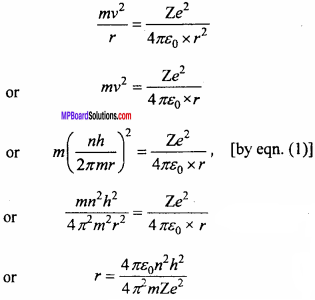In the above expression except n all the quantities are constant.
∴ r ∝ n2.

Question 4.
In the Balmer series of hydrogen spectrum the two lines are at 4102 Å and 4861Å, if the difference of wave number of these lines is same as the wave number of either spectral lines, then which series will be represented by this line? What will be the wavelength of this line?
Let n1 = 2 and n2 = x (for 4102 Å) and for line 4861 Å n1 = 2 and n2 = y.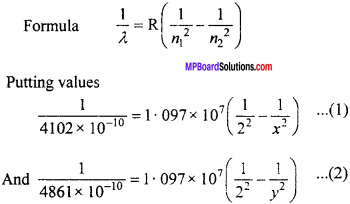Solving equation (1) and (2) we get,
x = 6 and y = 4
when the electron jumps from n2 = 6 to n1 = 4, then we get a third spectral line which belongs to Brackett’s series.
The wavelength of this spectral line be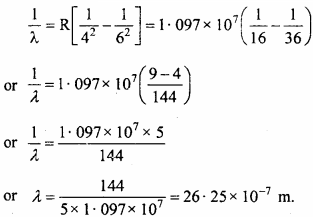Question 5.
State main postulates of Bohr’s atomic model.
The main postulates of Bohr’s atomic model are as follows :
1. Entire mass and entire positive charge of atom is concentrated at the nucleus.

2. The electrostatic attraction force between nucleus and electron provides the require centripetal force to electrons to revolve round the nucleus.

3. The electron does not revolve round the nucleus in all possible orbits, but they revolve in some specific orbits. These orbits are called as stable orbits.

4. The stable orbits are those orbits in which the angular momentum of electron is integral multiple of $$\frac {h}{2π}$$, where h is Plank constant (h = 6.63 x 10-34J-sec.).
If m is mass of electron r is radius of orbit and v is its speed then angular momentum
mvr = $$\frac {nh}{2π}$$, where n = 1, 2, 3,
n is called as principal quantum number.

5. The electrons revolving in stable orbits neither absorb nor emit the energy. The emission or absorption of energy takes place only when they jump from one orbit to other.

Atoms Numerical Questions

Question 1.
The ionization energy of hydrogen atom is 13.6 eV. An electron which jumps from ground state to n = 4 state. Calculate the wavelength of photon.
Solution:
Energy in ground state of hydrogen atom E1 = -136 eV
Energy in n = 4 state E4 = $$\frac { -13.6 }{ { 4 }^{ 2 } }$$ = – 0.85 eV
∴ Energy of photon = E4 – E1 = – 0.85 + 13.6 = 12.75 eV
∵ hν = E and c = νλ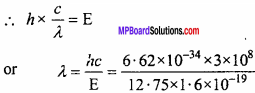⇒ λ = 973 x 10-10 m = 973 Å.Question 2.
If the value of energy for an impact parameter is increased then, will the scattering angle increase or decrease? (NCERT)
Solution: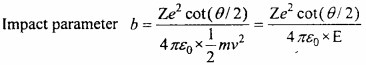If the energy is increased then in the same ratio, the value of cot(θ/2)should be increased i.e., the angle of scattering will decrease.

Question 3.
What should be the value of angle of scattering for impact parameter b = 0? (NCERT)
Solution:
We know thatIf b = 0, then cot(θ/2) = 0 = cot(90°)
⇒ $$\frac {θ}{2}$$ = 90°
or θ = 180°

Question 4.
In a Rutherford’s experiment the number of α – particle scattered at 90° is 28 per minute. Find out the number of particles scattered at 120°.
Solution: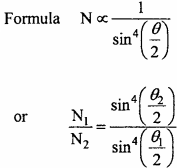Given θ1 = 90°, θ2 = 120° and N1 = 28 per minute
Putting values in the formula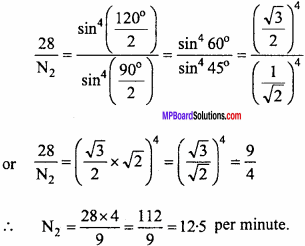Question 5.
Up to what least distant from the nucleus of silver (Z = 47) or α – particles of energy 8 MeV can reach?
Solution:
Formula : r0 = $$\frac { 1 }{ 4\pi { \varepsilon }_{ 0 } } .\frac { 2Z{ e }^{ 2 } }{ E }$$
Given : $$\frac { 1 }{ 4\pi { \varepsilon }_{ 0 } }$$ = 9 x 109 Nm2c-2, Z = 47, e = 1.6 x 10-19C
E = 8MeV = 8 x 106 x 1.6 x 10-19J
Putting values in the formula